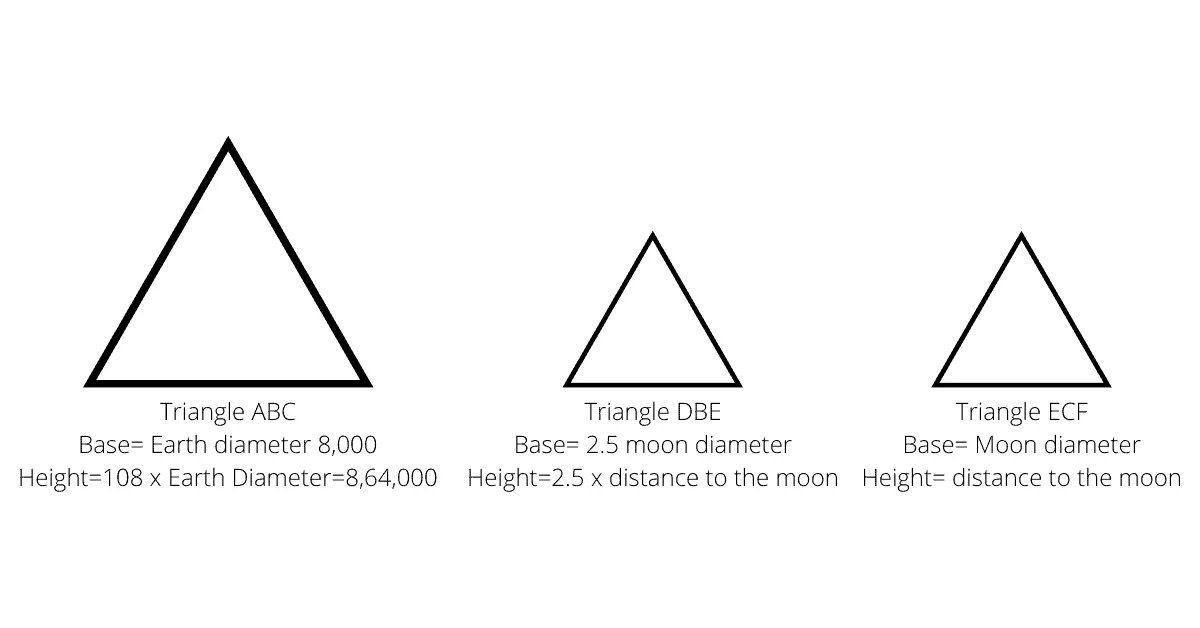# Measure the distance of the moon and its radiusOur natural satellite, the Moon, has been attracting people’s views for more than one millennium. It is the second brightest object in the sky after the Sun and in many ways has an impact on earthly life, for example, it is thanks to the Moon that there are ebb and flow. The distance to the moon was first measured by the ancient Greek astronomer and mathematician Hipparchus in the second century BC.

### Angular size of the moon

First, let’s decide on the input data that we need for calculations. During a total solar eclipse, we can see that the lunar disk almost perfectly overlaps the sun’s surface. This observation tells astronomers that the angular sizes of the Moon and the Sun are practically the same. Angular diameter refers to the angle between two rays emitted from the observer’s eyes that pass through the extreme opposite points of the measured object

You don’t need any special tools to take measurements. On a full moon, fold a small piece of paper so that it completely covers the moon’s disk. By dividing the width of the paper by the distance from it to your eyes, you get the angular size expressed in radians. In this case, there is no need to apply a mathematically exact formula, since for small angles tg α ≈ α . Don’t make such measurements for the Sun! You could seriously damage your eyes.

Determining the angular dimensions of distant objects and the angular distances between objects is an important part of astronomical observations and will be repeatedly mentioned in future materials. Minutes and arc seconds are usually used to indicate them. To convert minutes of arc to degrees, simply divide the value by 60, for example, the apparent diameter of the moon is approximately 30 ′ or 0.5 degrees.

The second, often used unit of measurement is radians, it allows you to simplify preliminary calculations and get rid of trigonometry. One radian is the angle that corresponds to an arc, the length of the radius of the circle (see figure). To convert minutes of arc to radians, the indicator needs to be multiplied by π / 10800 , so we get a value of ~ 0.0087 for the Moon.

We already know the approximate radius of the Earth from the previous article, and we also know about the existence of lunar eclipses, during which our planet casts a shadow on the surface of the Moon. For further calculations, we also need the angular size of the earth’s shadow in a total lunar eclipse. It is more than two and a half times the diameter of the moon and, accordingly, measuring the shadow directly is somewhat problematic.

However, in the course of observations, it is possible to detect the time during which the Moon will first be completely obscured by a shadow from one edge of the Earth, and then measure the time until the moment when the shadow from the opposite edge begins to leave the lunar disk. By solving the proportion, we get an approximate value of 80 ′ or 0.023 radians. Now that we have all the necessary input data, we can start calculating.

## Distance to the moon

All calculations are based on simple Euclidean geometry. We will base ourselves on the assumption that the distance between the Earth and the Sun is much greater than the distance to the Moon. Thus, we can consider the angle α equal to the angular diameter of the Sun, which, in turn, is approximately equal to the lunar one.

The diameter of the Earth is the base of triangle ABC , while the unknown extent of the shadow during the lunar eclipse is the base of A′BC ′ . These isosceles triangles are similar, since they have the same angles, therefore, the ratio of their heights is equal to the coefficient of similarity.

If we denote the distance to the Moon through L , then the diameter of the earth’s shadow will be equal to D ЗТ = L * β . Also, the height of the triangle A′BC ′ is equal to H Л = H З – L , and the height ABC is equal to H З = D З / α .

Multiplying the distance to the Moon by its angular size, we get an approximate diameter of 3497 km, which is very close to reality. For comparison, we present the exact modern data: the semi-major axis is 384 399 km, the average diameter is 3474 km. It turned out quite well given the low accuracy of angular measurements. You can calculate the diameter of the earth’s shadow yourself, we have already received all the data necessary for this.

At the moment we know that the Moon’s orbit is elliptical with an eccentricity of 0.0549. At its closest point (perigee), the satellite approaches us 356 400 km, and its maximum distance (apogee) is 406 700 km. The distance to the moon in our time is determined with fantastic accuracy using laser ranging. On July 21, 1969, the Apollo 11 astronauts left the first corner reflector on the lunar surface designed for this kind of measurement.

The essence of the method is that a focused laser beam is sent from the Earth to the reflector (on the lunar surface, the beam area is about 25 km 2 ), part of the light returns back to the detector. Knowing the exact time taken by light to travel there and back, as well as the speed of light, you can easily determine the distance.